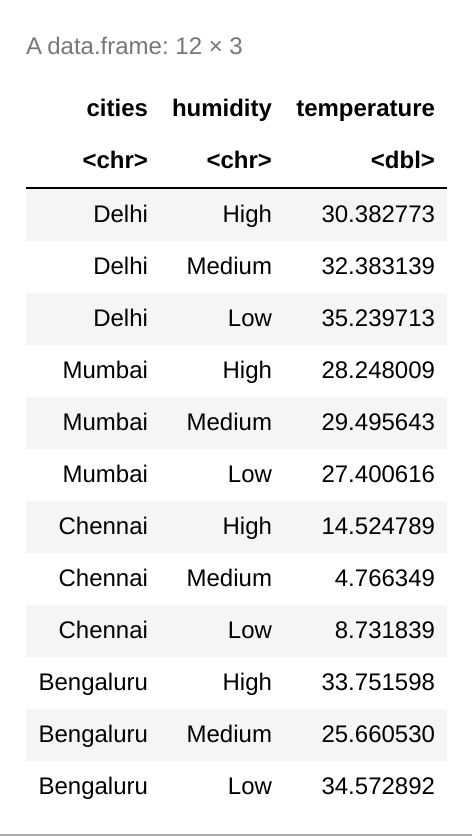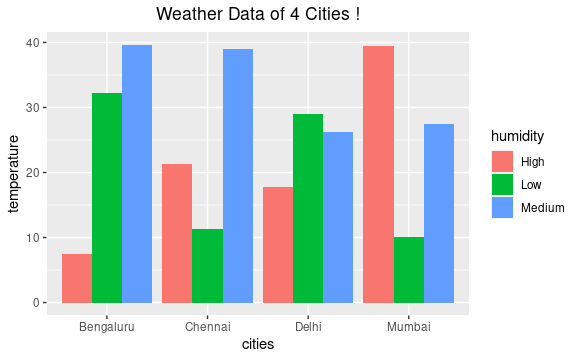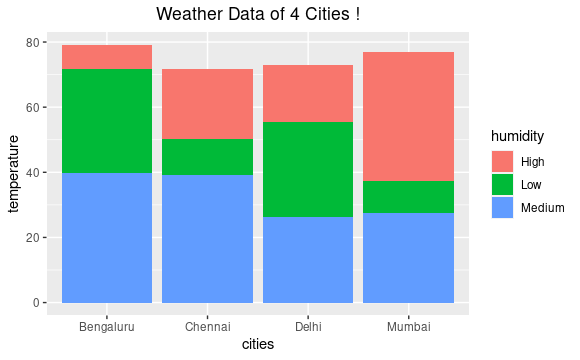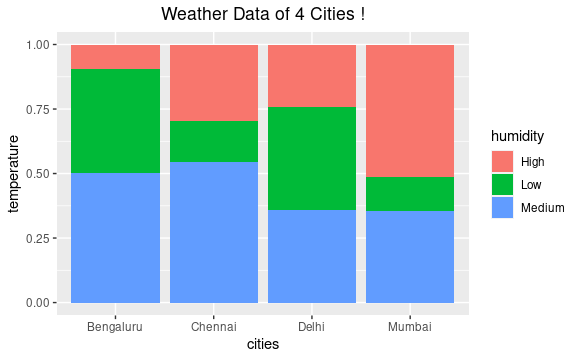Open In App

# Grouped, stacked and percent stacked barplot in ggplot2

The ggplot is a library used for generating graphs in R language. We provide the data and specify the aesthetics as to how the specified data should be mapped. It is a very powerful library and widely used to generate comprehensive graphs and plots. It is used for creating graphics based on the “Grammar of Graphics”.

A Bar Plot or Bar Chart is a Data Visualization tool that is widely used to represent the relationship between a numeric and a categorical variable. The numeric variable is generally plotted on the Y-axis and the categorical variable on the horizontal X-axis. The height of the bars represents the corresponding numeric value of the categorical value. The above-mentioned come in handy when we have more than one categorical variable and a numeric variable.

In this article, we will be seeing how we can plot 3 different types of Bar Plots. These 3 different types of Bar Plots are :

• Grouped Bar Plot
• Stacked Bar Plot
• Percent Stacked Bar Plot

The only difference in the codes of the 3 plots is the value of the “position” parameter in the geom_bar() function of the ggplot library. Given below is implementation of the same.

Example :

## R

 `# importing the ggplot2 library``library``(ggplot2)`` ` `# creating the cities column``# c() is used to combine vectors``# rep() is used for replication of values``cities <- ``c``(``rep``(``"Delhi"``, 3), ``rep``(``"Mumbai"``, 3),``            ``rep``(``"Chennai"``, 3), ``rep``(``"Bengaluru"``, 3))`` ` `# creating the humidity column``# contains 3 classes ``humidity <- ``rep``(``c``(``"High"``, ``"Medium"``, ``"Low"``), 4)`` ` `# creating the temperature column``# abs() is used for getting the absolute value``# rnorm() is used for generating random variates``# in a normal distribution``# rnorm(number of samples, mean, SD)``temperature <- ``abs``(``rnorm``(12, 25, 10))`` ` `# dataframe consisting of the three columns``dataframe <- ``data.frame``(cities, humidity,``                        ``temperature)`` ` `# calling the dataframe``dataframe`## Grouped Bar Plots

Grouped Bar Plots or Clustered Bar Plots are used to extend the functionalities of a single variate or single category bar plot to a multi variate bar plot. In these plots, the bars are grouped according to their categories and the colors are the differentiating factor to represent the other categorical variable. The bars are positioned catering to one group or the primary group and the colors represent the secondary category. For grouped bar plots, the value of position parameter is specified as “dodge”.

Approach:

• Import module
• Create dataframe
• Plot graph with required functions
• Set position parameter to dodge in geom_bar( ) function
• Display plot

Syntax :

geom_bar(position = “dodge” , ….)

Example:

## R

 `# importing the ggplot2 library``library``(ggplot2)`` ` `# creating data frame``cities <- ``c``(``rep``(``"Delhi"``, 3), ``rep``(``"Mumbai"``, 3),``            ``rep``(``"Chennai"``, 3), ``rep``(``"Bengaluru"``, 3))``  ` `humidity <- ``rep``(``c``(``"High"``, ``"Medium"``, ``"Low"``), 4)`` ` `temperature <- ``abs``(``rnorm``(12, 25, 10))`` ` `dataframe <- ``data.frame``(cities, humidity,``                        ``temperature)`` ` `# calling the dataframe``dataframe`` ` `# plotting the graph``ggplot``(dataframe, ``aes``(fill = humidity,``                      ``y = temperature, x = cities))+``geom_bar``(position = ``"dodge"``, stat = ``"identity"``)+``ggtitle``(``"Weather Data of 4 Cities !"``)+``theme``(plot.title = ``element_text``(hjust = 0.5))`

Output:Grouped Bar Plot for the Weather Data Set

## Stacked Bar Plots

Stacked Bar Plots or Stacked Bar Graphs are an extension of the standard Bar Plots wherein we can represent two categorical variables with the help of a single Bar Plot. In these plots, the bars of the primary categorical variable determine the position and the varying levels of the secondary categorical variable which are differentiated on the basis of their colors are stacked on top of each other. For stacked bar plots, value of the position parameter is specified as “stack”.

Approach:

• Import module
• Create dataframe
• Plot graph with required functions
• Set position parameter to stack in geom_bar( ) function
• Display plot

Syntax :

geom_bar(position = “stack” , …)

Example:

## R

 `# importing the ggplot2 library``library``(ggplot2)`` ` `# creating data frame``cities <- ``c``(``rep``(``"Delhi"``, 3), ``rep``(``"Mumbai"``, 3),``            ``rep``(``"Chennai"``, 3), ``rep``(``"Bengaluru"``, 3))`` ` `humidity <- ``rep``(``c``(``"High"``, ``"Medium"``, ``"Low"``), 4)`` ` `temperature <- ``abs``(``rnorm``(12, 25, 10))`` ` `dataframe <- ``data.frame``(cities, humidity,``                        ``temperature)`` ` `# calling the dataframe``dataframe`` ` `# plotting graph``ggplot``(dataframe, ``aes``(fill = humidity,``                      ``y = temperature, x = cities))+``geom_bar``(position = ``"stack"``, stat = ``"identity"``)+``ggtitle``(``"Weather Data of 4 Cities !"``)+``theme``(plot.title = ``element_text``(hjust = 0.5))`

Output:Stacked Bar Plot of the Weather Data Set

## Percent Stacked Bar Plots

The Percent Stacked Bar Plots are used to visualize the contribution or proportion of each categorical variable in while cumulatively taking the primary categorical variable. The entire bar is filled to the top and the different groups occupy the heights corresponding to their proportion in the bar. To map a percent stacked bar plot, the value of the position parameter is specified as “fill”.

Approach:

• Import module
• Create dataframe
• Plot graph with required functions
• Set position parameter to fill in geom_bar( ) function
• Display plot

Syntax :

geom_bar(position = “fill” , ….)

Example:

## R

 `# importing the ggplot2 library``library``(ggplot2)`` ` `# creating data frame``cities <- ``c``(``rep``(``"Delhi"``, 3), ``rep``(``"Mumbai"``, 3),``            ``rep``(``"Chennai"``, 3), ``rep``(``"Bengaluru"``, 3))`` ` `humidity <- ``rep``(``c``(``"High"``, ``"Medium"``, ``"Low"``), 4)`` ` `temperature <- ``abs``(``rnorm``(12, 25, 10))`` ` `dataframe <- ``data.frame``(cities, humidity,``                        ``temperature)`` ` `# calling the dataframe``dataframe`` ` `# plotting graph``ggplot``(dataframe, ``aes``(fill = humidity,``                      ``y = temperature, x = cities))+``geom_bar``(position = ``"fill"``, stat = ``"identity"``)+``ggtitle``(``"Weather Data of 4 Cities !"``)+``theme``(plot.title = ``element_text``(hjust = 0.5))`

Output :Percent Stacked Bar Plot of Weather Data Set